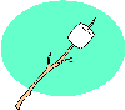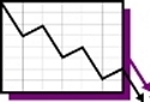,
Lesson

# Utility

Updated: December 14 2015,

### Concepts

Can happiness be measured? Students will learn how utility relates to economic decision making and the law of diminishing marginal utility.

### Introduction

Does man seek pleasure and avoid pain? Do consumers seek to maximize pleasure in their pursuits? Economists use the term utility to describe the satisfaction consumers receive from consumption. Students, will try to measure and graph total and marginal utility. (Utility does not explain why some people volunteer to work in a homeless shelter or give millions away to an orphanage, but provides a reasonable explanation of how humans try to maximize pleasure. Some behavior is outside the scope of economics such as self-destructive behavior. The lesson shows how rational consumers behave.)

### Learning Objectives

• Learn how behavior is linked to utility.
• Graph a total utility curve.
• Graph a marginal utility curve.
• Observe diminishing marginal utility.
• Equate marginal utility with marginal cost.
• Determine a connection between marginal utility and the law of demand.

### Resource List

• Utility Worksheet: This worksheet contains problems for students to complete plus the extension activity.
Utility Worksheet

• Utility Solutions: Solutions are provided on this sheet regarding the information from the "Utility" worksheet.
Utility Solutions

• How to Calculate Marginal Revenue Article:  For additional help this article provides a definition of marginal revenue and provides an example of how to calculate marginal revenue through 5 steps.
www.ehow.com/how_5144961_calculate-marginal-revenue.html

• Diminishing Marginal Utility: This is a YouTube video explaining the concept of diminishing marginal utility. (Note: If YouTube is blocked at your school, we suggest that you download the video ahead of time, or simply repeat the experiment in your own classroom.)

### Process

[Note: For additional information on how to calculate marginal revenue  read the following article ]

1. Get a large bag of jet puffed marshmallows. (I prefer Campfire Giant marshmallows.)2. Introduce the idea of utility (satisfaction) and ask for a volunteer.

3. If there are too many volunteers, have them choose a number between one and 100. Chose the student closest to your number. You can use a TI-83 to pick a random number for you.

4. Place a desk or chair in the front of the room facing the class and have the student sit in it.

5. Explain what utility means to the entire class.

6. Draw a graph on the board, labeling the Y-axis 'Utility' and the X-axis 'Number of Marshmallows Consumed'.

7. Place the numbers 1 to 10 on the vertical side of the graph.

8. Explain to the volunteer that he or she is to eat one marshmallow and then rate the utility they receive from 1 to 10. (10 is the highest utility)

9. After each marshmallow is consumed, have the volunteer rate his or her satisfaction and place a point on the graph that represents this.

10. Continue until the student's satisfaction begins to drop. It is up to you how far you want this drop to go to prove the law.(This should take about 15 minutes.)

11. Using the graph that you have drawn on the board, show how to calculate the slope of the total utility curve. Explain to the class that this is marginal utility.  To calculate the slope of a curve remember: “Rise over run makes finding the slope fun.”  So take the change in Y divided by the change in X.  You should find that the change gets smaller with each increment in X.

12. Draw another graph, and plot marginal utility. Explain that the marginal utility curve slopes down and to the right just like the demand curve. Explain that as more marshmallows are consumed, the consumer receives less and less satisfaction. In economics, this is called diminishing marginal utility. (Here is the author’s YouTube video on Diminishing Marginal Utility . If YouTube is blocked at your school, we suggest that you download the video ahead of time.)

13. Explain how economists use marginal analysis to equate marginal utility with marginal cost.

14. On your graph of marginal utility, show how many marshmallows would be consumed if the price where \$2. (Assume that the price is constant.)

15. Repeat step 14 but lower the price. (The students should observe that more is consumed.) Explain that this is the law of demand.

16. Ask if diminishing marginal utility applies to the consumption of all goods. (It should.)

17. Ask how decision making with marginal analysis could be used to determine how long to study or workout.

18. Distribute the homework assignment, Utility. For solutions to the worksheet look at Utility Solutions.

### Conclusion

Ask the students how the graphs might have changed if a different student would have been selected. (A different student would have a different utility). Economists do not have a way of measuring utility, but utility is a useful way to make predictions about behavior. In economics, it is assumed that consumers make consistent rational decisions and seek to maximize their utility with a fixed income. (The choice of utility units is arbitrary. I have used money as a standard to measure utility, but other measures could have been used.) Ask the students why they continued to eat marshmallows even though their utility was declining.  (Students will weigh the benefits of eating one more marshmallow with the cost.  As long as the benefit is greater than the cost, the student will continue to eat another marshmallow.  This is marginal analysis.)

### Extension Activity

In this activity you will see how to make decisions on the margin using your TI-83 Graphing calculator. Suppose that marginal utility for rock polishers is given by the formula,Y1=iPart((1/(2X^.5)*100)). Suppose the price of rock polishers is given by the formula, Y2=25. Key these formulas into your calculator and graph. Using your table function, verify that four rock polishers would be purchased? Now change the price in Y2 to 18. How many rock polishers would be purchased now?  Explain how marginal utility proves the law of demand. [When the price is reduced from \$25 to \$18, the quantity demanded increased from 4 to 7. This proves the law of demand since the quantity demanded increased as the price decreased.]

### Assessment

Use the following information to graph the total utility and marginal utility for Juan’s consumption of chocolate covered strawberries. Q=0 TU=0; Q=1 TU=9 Q=2 TU=14; Q=4 TU=18; Q=5 TU=21; Q=6 TU=23; Q=7 TU=24